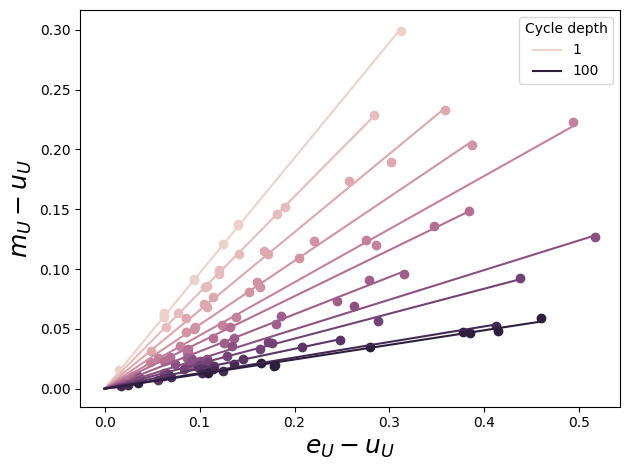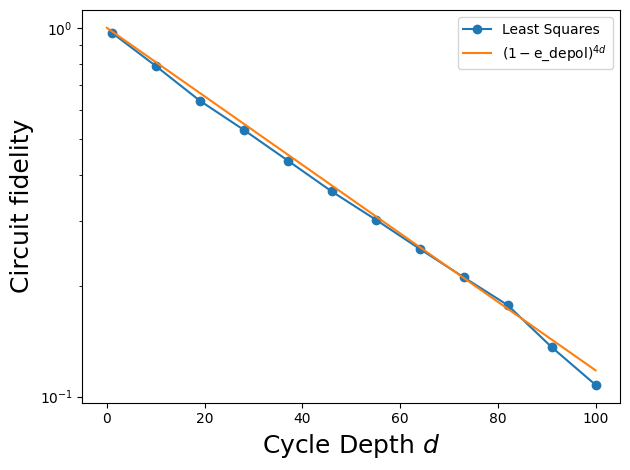# Cross Entropy Benchmarking Theory

try:
import cirq
except ImportError:
print("installing cirq...")
!pip install --quiet cirq
import cirq
print("installed cirq.")


Cross entropy benchmarking uses the properties of random quantum programs to determine the fidelity of a wide variety of circuits. When applied to circuits with many qubits, XEB can characterize the performance of a large device. When applied to deep, two-qubit circuits it can be used to accurately characterize a two-qubit interaction potentially leading to better calibration.

# Standard imports
import numpy as np

from cirq.contrib.svg import SVGCircuit


## The action of random circuits with noise

An XEB experiment collects data from the execution of random circuits subject to noise. The effect of applying a random circuit with unitary $$U$$ is modeled as $$U$$ followed by a depolarizing channel. The result is that the initial state $$|𝜓⟩$$ is mapped to a density matrix $$ρ_U$$ as follows:

$|𝜓⟩ → ρ_U = f |𝜓_U⟩⟨𝜓_U| + (1 - f) I / D$

where $$|𝜓_U⟩ = U|𝜓⟩$$, $$D$$ is the dimension of the Hilbert space, $$I / D$$ is the maximally mixed state, and $$f$$ is the fidelity with which the circuit is applied.

For this model to be accurate, we require $$U$$ to be a random circuit that scrambles errors. In practice, we use a particular circuit ansatz consisting of random single-qubit rotations interleaved with entangling gates.

### Possible single-qubit rotations

Geometrically, we choose 8 axes in the XY plane to perform a quarter-turn (pi/2 rotation) around. This is followed by a rotation around the Z axis of 8 different magnitudes.

These 8*8 possible rotations are chosen randomly when constructing the circuit.

exponents = np.linspace(0, 7/4, 8)
exponents

array([0.  , 0.25, 0.5 , 0.75, 1.  , 1.25, 1.5 , 1.75])

import itertools
SINGLE_QUBIT_GATES = [
cirq.PhasedXZGate(x_exponent=0.5, z_exponent=z, axis_phase_exponent=a)
for a, z in itertools.product(exponents, repeat=2)
]
SINGLE_QUBIT_GATES[:10], '...'

([cirq.PhasedXZGate(axis_phase_exponent=0.0, x_exponent=0.5, z_exponent=0.0),
cirq.PhasedXZGate(axis_phase_exponent=0.0, x_exponent=0.5, z_exponent=0.25),
cirq.PhasedXZGate(axis_phase_exponent=0.0, x_exponent=0.5, z_exponent=0.5),
cirq.PhasedXZGate(axis_phase_exponent=0.0, x_exponent=0.5, z_exponent=0.75),
cirq.PhasedXZGate(axis_phase_exponent=0.0, x_exponent=0.5, z_exponent=1.0),
cirq.PhasedXZGate(axis_phase_exponent=0.0, x_exponent=0.5, z_exponent=1.25),
cirq.PhasedXZGate(axis_phase_exponent=0.0, x_exponent=0.5, z_exponent=1.5),
cirq.PhasedXZGate(axis_phase_exponent=0.0, x_exponent=0.5, z_exponent=1.75),
cirq.PhasedXZGate(axis_phase_exponent=0.25, x_exponent=0.5, z_exponent=0.0),
cirq.PhasedXZGate(axis_phase_exponent=0.25, x_exponent=0.5, z_exponent=0.25)],
'...')


### Random circuit

We use cirq.experiments.random_quantum_circuit_generation.random_rotations_between_two_qubit_circuit to generate a random two-qubit circuit. Note that we provide the possible single-qubit rotations from above and declare that our two-qubit operation is the $$\sqrt{i\mathrm{SWAP} }$$ gate.

import cirq_google as cg
from cirq.experiments import random_quantum_circuit_generation as rqcg

q0, q1 = cirq.LineQubit.range(2)
circuit = rqcg.random_rotations_between_two_qubit_circuit(
q0, q1,
depth=4,
two_qubit_op_factory=lambda a, b, _: cirq.SQRT_ISWAP(a, b),
single_qubit_gates=SINGLE_QUBIT_GATES
)
SVGCircuit(circuit)

findfont: Font family 'Arial' not found.## Estimating fidelity

Let $$O_U$$ be an observable that is diagonal in the computational basis. Then the expectation value of $$O_U$$ on $$ρ_U$$ is given by

$Tr(ρ_U O_U) = f ⟨𝜓_U|O_U|𝜓_U⟩ + (1 - f) Tr(O_U / D).$

This equation shows how $$f$$ can be estimated, since $$Tr(ρ_U O_U)$$ can be estimated from experimental data, and $$⟨𝜓_U|O_U|𝜓_U⟩$$ and $$Tr(O_U / D)$$ can be computed.

Let $$e_U = ⟨𝜓_U|O_U|𝜓_U⟩$$, $$u_U = Tr(O_U / D)$$, and $$m_U$$ denote the experimental estimate of $$Tr(ρ_U O_U)$$. We can write the following linear equation (equivalent to the expression above):

$m_U = f e_U + (1-f) u_U \\ m_U - u_U = f (e_U - u_U)$

# Make long circuits (which we will truncate)
MAX_DEPTH = 100
N_CIRCUITS = 10
circuits = [
rqcg.random_rotations_between_two_qubit_circuit(
q0, q1,
depth=MAX_DEPTH,
two_qubit_op_factory=lambda a, b, _: cirq.SQRT_ISWAP(a, b),
single_qubit_gates=SINGLE_QUBIT_GATES)
for _ in range(N_CIRCUITS)
]

# We will truncate to these lengths
cycle_depths = np.arange(1, MAX_DEPTH + 1, 9)
cycle_depths

array([  1,  10,  19,  28,  37,  46,  55,  64,  73,  82,  91, 100])


### Execute circuits

Cross entropy benchmarking requires sampled bitstrings from the device being benchmarked as well as the true probabilities from a noiseless simulation. We find these quantities for all (cycle_depth, circuit) permutations.

pure_sim = cirq.Simulator()

# Pauli Error. If there is an error, it is either X, Y, or Z
# with probability E_PAULI / 3
E_PAULI = 5e-3
noisy_sim = cirq.DensityMatrixSimulator(noise=cirq.depolarize(E_PAULI))

# These two qubit circuits have 2^2 = 4 probabilities
DIM = 4

records = []
for cycle_depth in cycle_depths:
for circuit_i, circuit in enumerate(circuits):

# Truncate the long circuit to the requested cycle_depth
circuit_depth = cycle_depth * 2 + 1
assert circuit_depth <= len(circuit)
trunc_circuit = circuit[:circuit_depth]

# Pure-state simulation
psi = pure_sim.simulate(trunc_circuit).final_state_vector
pure_probs = np.abs(psi)**2

# Noisy execution
meas_circuit = trunc_circuit + cirq.measure(q0, q1)
sampled_inds = noisy_sim.sample(meas_circuit, repetitions=10_000).values[:,0]
sampled_probs = np.bincount(sampled_inds, minlength=DIM) / len(sampled_inds)

# Save the results
records += [{
'circuit_i': circuit_i,
'cycle_depth': cycle_depth,
'circuit_depth': circuit_depth,
'pure_probs': pure_probs,
'sampled_probs': sampled_probs,
}]
print('.', end='', flush=True)

........................................................................................................................


## What's the observable

What is $$O_U$$? Let's define it to be the observable that gives the sum of all probabilities, i.e.

$O_U |x \rangle = p(x) |x \rangle$

for any bitstring $$x$$. We can use this to derive expressions for our quantities of interest.

$e_U = \langle \psi_U | O_U | \psi_U \rangle \\ = \sum_x a_x^* \langle x | O_U | x \rangle a_x \\ = \sum_x p(x) \langle x | O_U | x \rangle \\ = \sum_x p(x) p(x)$

$$e_U$$ is simply the sum of squared ideal probabilities. $$u_U$$ is a normalizing factor that only depends on the operator. Since this operator has the true probabilities in the definition, they show up here anyways.

$u_U = \mathrm{Tr}[O_U / D] \\ = 1/D \sum_x \langle x | O_U | x \rangle \\ = 1/D \sum_x p(x)$

For the measured values, we use the definition of an expectation value

$\langle f(x) \rangle_\rho = \sum_x p(x) f(x)$

It becomes notationally confusing because remember: our operator on basis states returns the ideal probability of that basis state $$p(x)$$. The probability of observing a measured basis state is estimated from samples and denoted $$p_\mathrm{est}(x)$$ here.

$m_U = \mathrm{Tr}[\rho_U O_U] \\ = \langle O_U \rangle_{\rho_U} = \sum_{x} p_\mathrm{est}(x) p(x)$

for record in records:
e_u = np.sum(record['pure_probs']**2)
u_u = np.sum(record['pure_probs']) / DIM
m_u = np.sum(record['pure_probs'] * record['sampled_probs'])
record.update(
e_u=e_u,
u_u=u_u,
m_u=m_u,
)


Remember:

$m_U - u_U = f (e_U - u_U)$

We estimate f by performing least squares minimization of the sum of squared residuals

$\sum_U \left(f (e_U - u_U) - (m_U - u_U)\right)^2$

over different random circuits. The solution to the least squares problem is given by

$f = (∑_U (m_U - u_U) * (e_U - u_U)) / (∑_U (e_U - u_U)^2)$

import pandas as pd
df = pd.DataFrame(records)
df['y'] = df['m_u'] - df['u_u']
df['x'] = df['e_u'] - df['u_u']

df['numerator'] = df['x'] * df['y']
df['denominator'] = df['x'] ** 2


### Fit

We'll plot the linear relationship and least-squares fit while we transform the raw DataFrame into one containing fidelities.

%matplotlib inline
from matplotlib import pyplot as plt

# Color by cycle depth
import seaborn as sns
colors = sns.cubehelix_palette(n_colors=len(cycle_depths))
colors = {k: colors[i] for i, k in enumerate(cycle_depths)}

_lines = []
def per_cycle_depth(df):
fid_lsq = df['numerator'].sum() / df['denominator'].sum()

cycle_depth = df.name
xx = np.linspace(0, df['x'].max())
l, = plt.plot(xx, fid_lsq*xx, color=colors[cycle_depth])
plt.scatter(df['x'], df['y'], color=colors[cycle_depth])

global _lines
_lines += [l] # for legend
return pd.Series({'fidelity': fid_lsq})

fids = df.groupby('cycle_depth').apply(per_cycle_depth).reset_index()
plt.xlabel(r'$e_U - u_U$', fontsize=18)
plt.ylabel(r'$m_U - u_U$', fontsize=18)
_lines = np.asarray(_lines)
plt.legend(_lines[[0,-1]], cycle_depths[[0,-1]], loc='best', title='Cycle depth')
plt.tight_layout()### Fidelities

plt.plot(
fids['cycle_depth'],
fids['fidelity'],
marker='o',
label='Least Squares')

xx = np.linspace(0, fids['cycle_depth'].max())

# In XEB, we extract the depolarizing fidelity, which is
# related to (but not equal to) the Pauli error.
# For the latter, an error involves doing X, Y, or Z with E_PAULI/3
# but for the former, an error involves doing I, X, Y, or Z with e_depol/4
e_depol = E_PAULI / (1 - 1/DIM**2)

# The additional factor of four in the exponent is because each layer
# involves two moments of two qubits (so each layer has four applications
# of a single-qubit single-moment depolarizing channel).
plt.plot(xx, (1-e_depol)**(4*xx), label=r'$(1-\mathrm{e\_depol})^{4d}$')

plt.ylabel('Circuit fidelity', fontsize=18)
plt.xlabel('Cycle Depth $d$', fontsize=18)
plt.legend(loc='best')
plt.yscale('log')
plt.tight_layout()from cirq.experiments.xeb_fitting import fit_exponential_decays

# Ordinarily, we'd use this function to fit curves for multiple pairs.
# We add our qubit pair as a column.
fids['pair'] = [(q0, q1)] * len(fids)

fit_df = fit_exponential_decays(fids)
fit_row = fit_df.iloc
print(f"Noise model fidelity: {(1-e_depol)**4:.3e}")
print(f"XEB layer fidelity:   {fit_row['layer_fid']:.3e} +- {fit_row['layer_fid_std']:.2e}")

Noise model fidelity: 9.788e-01
XEB layer fidelity:   9.789e-01 +- 2.25e-04

[{ "type": "thumb-down", "id": "missingTheInformationINeed", "label":"Missing the information I need" },{ "type": "thumb-down", "id": "tooComplicatedTooManySteps", "label":"Too complicated / too many steps" },{ "type": "thumb-down", "id": "outOfDate", "label":"Out of date" },{ "type": "thumb-down", "id": "samplesCodeIssue", "label":"Samples / code issue" },{ "type": "thumb-down", "id": "otherDown", "label":"Other" }]
[{ "type": "thumb-up", "id": "easyToUnderstand", "label":"Easy to understand" },{ "type": "thumb-up", "id": "solvedMyProblem", "label":"Solved my problem" },{ "type": "thumb-up", "id": "otherUp", "label":"Other" }]